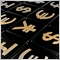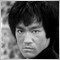• 概要
• レビュー (2)
• コメント (1)
• 最新情報

# OHLC Moving MTF Average

A truly advanced moving average indicator that replaces the stock MA indicator.

Fully customizable for advanced & unique moving average calculations.

Shows an initial setting of a moving OHLC average calculation by default.

### Key Features

• This indicator has a four moving average calculation with a sum divider.
• Each moving average has its own settings (except timeframe & ATR).
• Bar shift that takes prices from historical data (less repaint?).
• Price multiplier per moving average and a divider for all prices.
• ATR to adjust the prices higher or lower.

### Input Parameters

• MA TimeFrame = 0; Timeframe of Moving Average.
• MA Colorful = true; Customizable two color moving average line.
• MA Price Divider = 4; Sum divider of four moving averages.
• ATR Period = 0; 0:Off, or ATR for added MA price positioning.
• ATR Price Method = 1; 0:ATR added(UP), 1:ATR subtracted(DN).
• MA1 Period (# of Periods) = 14; Periods of Moving Average calculation.
• MA1 Shift (# of Periods) = 0; Periods of Moving Average shift.
• MA1 Method = 0; 0:Simple, Exponential, Smoothed, Linear Weighted.
• MA1 Price = 0; 0:Close, Open, High, Low, Median, Typical, Weighted.
• MA1 Price Multiple = 1; Must be 1 or higher.
• MA1 Bars Back = 0; 0: Current bar, reads historical bars.
• MA2 Period (# of Periods) = 14; Periods of Moving Average calculation.
• MA2 Shift (# of Periods) = 0; Periods of Moving Average shift.
• MA2 Method = 0; 0:Simple, Exponential, Smoothed, Linear Weighted.
• MA2 Price = 1; 0:Close, Open, High, Low, Median, Typical, Weighted.
• MA2 Price Multiple = 1; Must be 1 or higher.
• MA2 Bars Back = 0; 0: Current bar, reads historical bars.
• MA3 Period (# of Periods) = 14; Periods of Moving Average calculation.
• MA3 Shift (# of Periods) = 0; Periods of Moving Average shift.
• MA3 Method = 0; 0:Simple, Exponential, Smoothed, Linear Weighted.
• MA3 Price = 2; 0:Close, Open, High, Low, Median, Typical, Weighted.
• MA3 Price Multiple = 1; Must be 1 or higher.
• MA3 Bars Back = 0; 0: Current bar, reads historical bars.
• MA4 Period (# of Periods) = 14; Periods of Moving Average calculation.
• MA4 Shift (# of Periods) = 0; Periods of Moving Average shift.
• MA4 Method = 0; 0:Simple, Exponential, Smoothed, Linear Weighted.
• MA4 Price = 3; 0:Close, Open, High, Low, Median, Typical, Weighted.
• MA4 Price Multiple = 1; Must be 1 or higher.
• MA4 Bars Back = 0; 0: Current bar, reads historical bars.

### Integration

• There is only one buffer, of 0, which presents a value of MTF-OHLC-MA.

### Improvements

• Please be sure to leave any ideas, tips and suggestions in the "Reviews" section and they may be included.
• Improvements to the system could be at any time so we suggest that you possibly backup your copies.

おすすめの製品
フィルタ:1180
2019.06.15 10:38

Nice attempt. Bit too simple to call advanced.5517
2018.03.14 03:19

average indicator

バージョン 4.0 2018.03.23